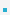5. Stacks and Queues

5.1 StackA stack is a last in, first out (LIFO) abstract data type and data structure.A stack is characterized by only three fundamental operations: push, pop and stack top.
– The push operation adds a new item to the top of the stack, or initializes the stack if it is empty. If    the stack is full and does not contain enough space to accept the given item, the stack is then    considered to be in an overflow state.
– The pop operation removes an item from the top of the stack. If the stack is empty then it goes into    underflow state.
– The stack top operation gets the data from the top-most position and returns it to the user without    deleting it. The same underflow state can also occur in stack top operation if stack is empty.A stack can be easily implemented either through an array or a linked list.

Implementation: ArrayThe array implementation aims to create an array where the first element (usually at the zero-offset) is    the bottom. That is, array is the first element pushed onto the stack and the last element popped    off.

 #include #include #define STACKSIZE 10 typedef struct {     int size;     int items[STACKSIZE]; } STACK; void push(STACK *ps, int x) {     if (ps->size == STACKSIZE) {         fputs("Error: stack overflow\n", stderr);         exit(0);     }     else         ps->items[ps->size++] = x; } int pop(STACK *ps) {     if (ps->size == 0){         fputs("Error: stack underflow\n", stderr);         exit(0);     }     else         return ps->items[--ps->size]; } int main() {     STACK list;     int i;     list.size = 0;     for (i=0; iThe linked-list implementation is equally simple and straightforward. In fact, a simple singly linked list    is sufficient to implement a stack.

5.2 QueueA queue is a first in, first out (FIFO) abstract data type and data structure.A queue is characterized by two fundamental operations: enqueue and dequeue.Queue overflow results from trying to add an element onto a full queue and queue underflow    happens when trying to remove an element from an empty queue.A queue can be easily implemented either through an array or a linked list.